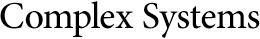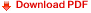The Effects of Boundary Conditions on Cellular AutomataBrian J. LuValle
Algorithmic Nature Group
LABORES for the Natural and Digital Sciences
Paris, France

Abstract

In this paper, we explore how the behavior of cellular automata (CAs) is affected by different boundary conditions and what types of systems it is affected by. Six experiments are presented. The first determines the most effective boundary condition for optimizing rule-output (in)equality related to Shannon entropy. The second experiment determines the effect of the boundary conditions compared to the size of the initial conditions. The third experiment determines the variation of systems for a specific Li–Packard and Wolfram class. The fourth experiment generalizes the principles of the third experiment into a rule space involving four binary cells rather than three. The fifth experiment determines the change in complexity of systems with different boundary conditions. Finally, the sixth experiment generalizes the concepts of the fifth experiment into the same rule space used in experiment four. Most of the time, boundary conditions do not change the classification, thus do not change the behavior. But some boundary conditions have been found to change the qualitative behavior. However, it is only constant boundary conditions that have been found to change the complexity of a system, and the constant boundary conditions only decrease the complexity. The largest change in the compression ratio was found to be for rule 110 when the boundary conditions change from cyclical to constant. The degree of this change is approximately 25%. Distributed and cyclical boundary conditions can be relied upon to maximize the rule-output equality of systems in general, while null boundary conditions can be relied upon to increase the overall complexity of a system.

Keywords: boundary conditions; Wolfram classes; Li–Packard class; predicting cellular automaton behavior; uncompressibility